# Real OCA Java SE 8 Programmer I 1Z0-808 Dumps Questions

Are you still looking for valid 1Z0-808 exam dumps? We have released real OCA Java SE 8 Programmer I 1Z0-808 Dumps Questions V11.02 to ensure that you can pass 1Z0-808 Java SE 8 Programmer I exam. Oracle 1Z0-808 dumps questions that help you to get fully prepared for the Oracle 1Z0-808 Java SE 8 Programmer I exam. DumpsBase provides all the necessary things to pass Oracle Java SE 8 Programmer 1Z0-808 exam with money back guarantee that reflects the confidence for Real OCA Java SE 8 Programmer I 1Z0-808 Dumps Questions.

## Free Dumps Online for 1Z0-808 Real Dumps Questions

1. Given: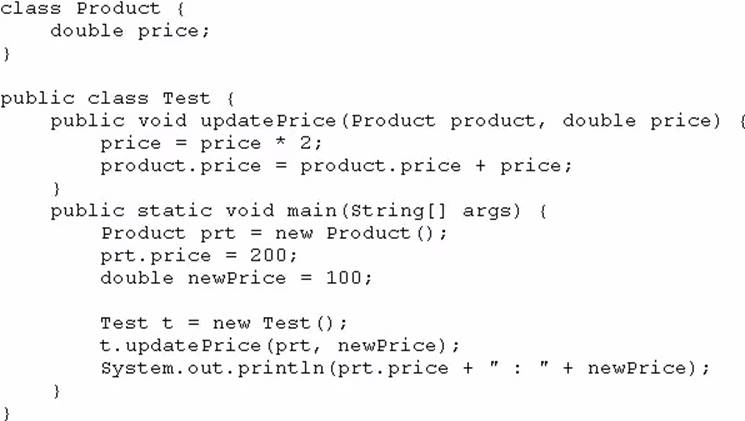What is the result?

2. Given the following segment of code: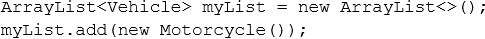Which two statements, if either were true, would make the code compile? (Choose two.)

3. Given the code fragment: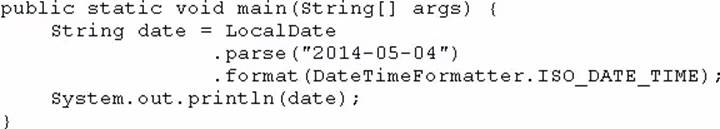What is the result?

4. Given the code fragment: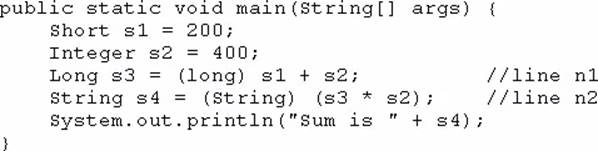What is the result?

5. What is the name of the Java concept that uses access modifiers to protect variables and hide them within a class?

6. Given the code fragment: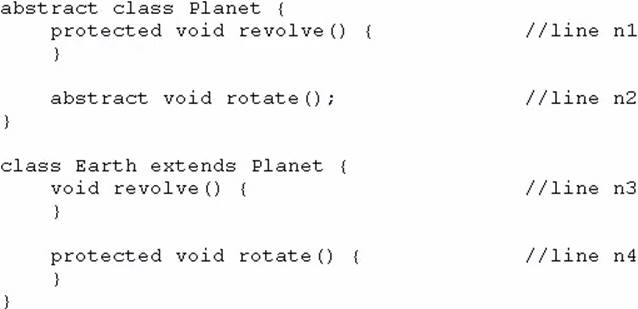Which two modifications, made independently, enable the code to compile? (Choose two.)

7. Given: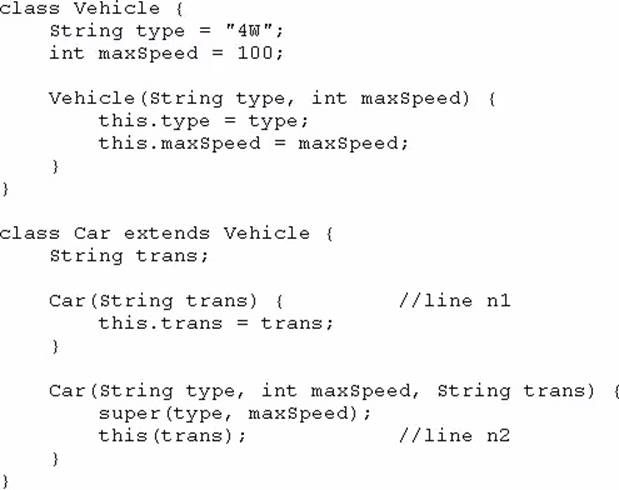And given the code fragment: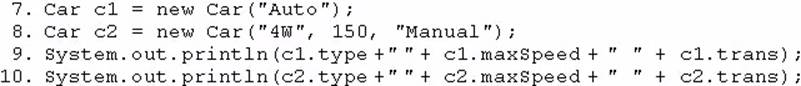What is the result?

8. Given the code fragment: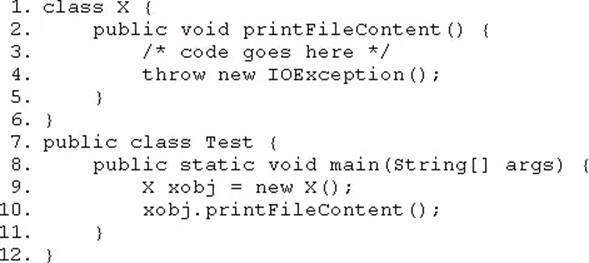Which two modifications should you make so that the code compiles successfully? (Choose two.)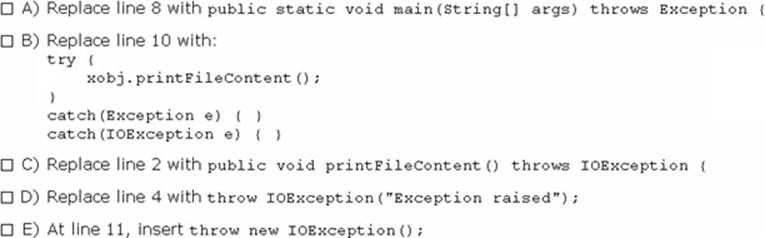9. Given the following two classes: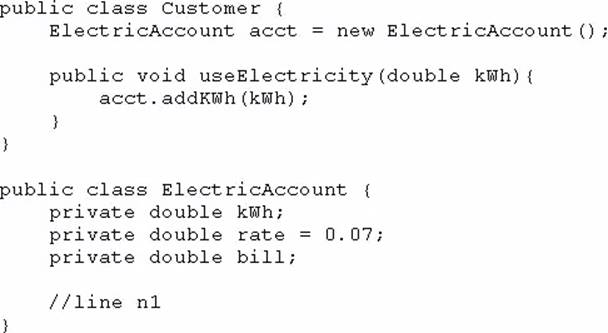How should you write methods in the ElectricAccount class at line n1 so that the member variable bill is always equal to the value of the member variable kwh multiplied by the member variable rate?

Any amount of electricity used by a customer (represented by an instance of the customer class) must contribute to the customer’s bill (represented by the member variable bill) through the method use Electricity method. An instance of the customer class should never be able to tamper with or decrease the value of the member variable bill.

10. Given the code fragment: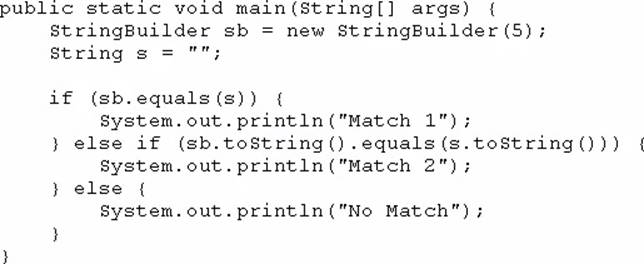What is the result?

11. Given: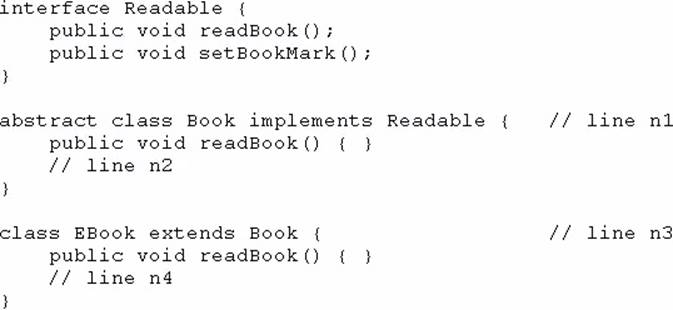And given the code fragment:

Book book1 = new EBook ();

Which option enables the code to compile?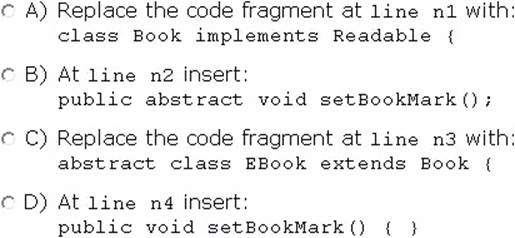12. Given: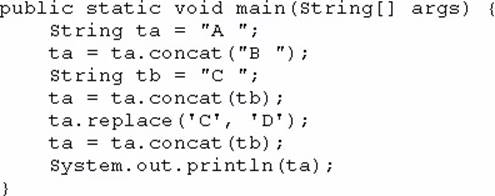What is the result?

13. Given: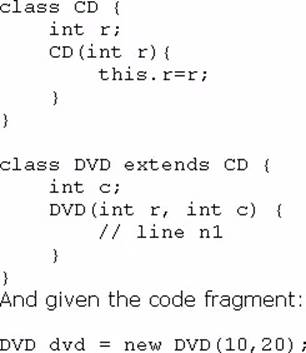Which code fragment should you use at line n1 to instantiate the dvd object successfully?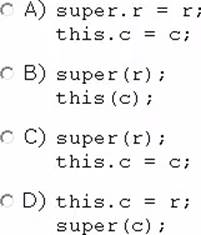14. Given the code fragment: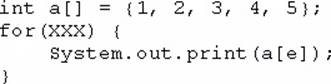Which option can replace xxx to enable the code to print 135?

15. Which statement best describes encapsulation?

16. Given the code fragment from three files: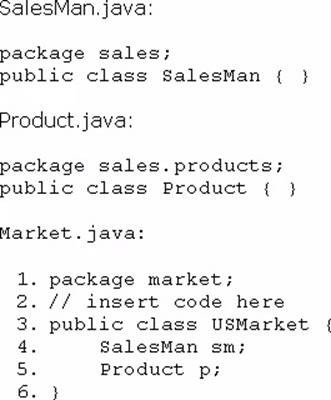Which code fragment, when inserted at line 2, enables the code to compile?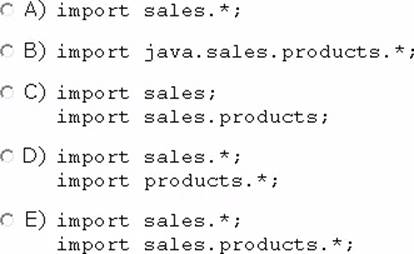17. Given the following class: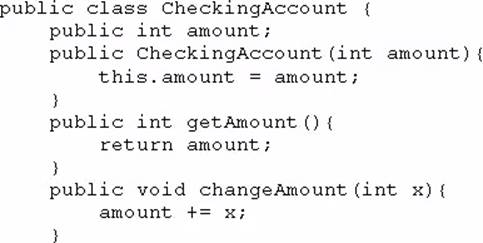And given the following main method, located in another class: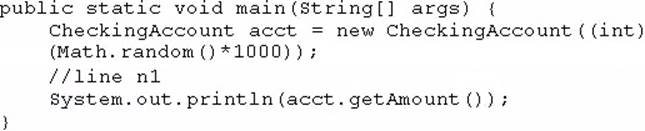Which three lines, when inserted independently at line n1, cause the program to print a 0 balance? (Choose three.)

18. Given the code fragment: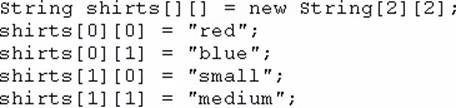Which code fragment prints red: blue: small: medium?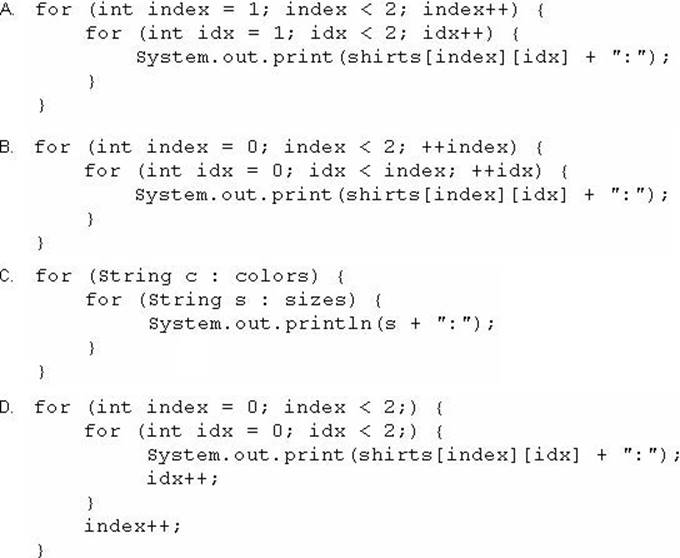19. Given the code fragment: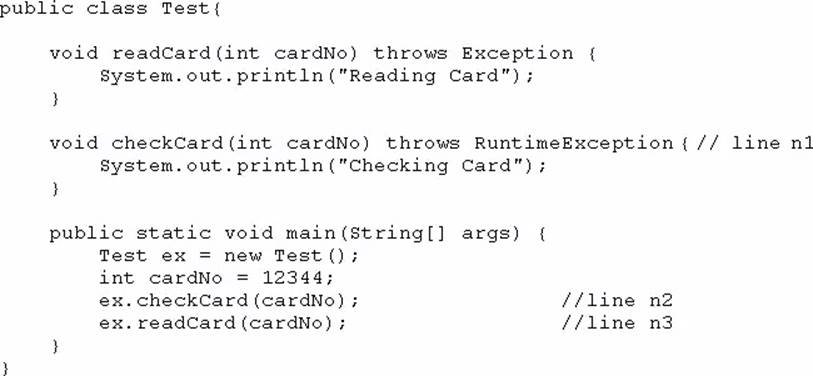What is the result?

20. Given the code fragment: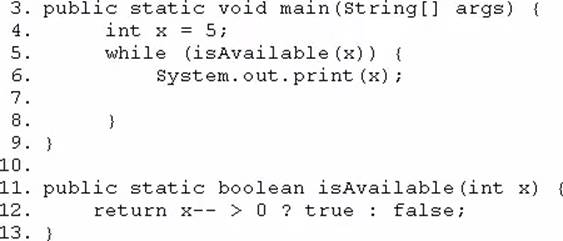Which modification enables the code to print 54321?

21. Given the code fragment: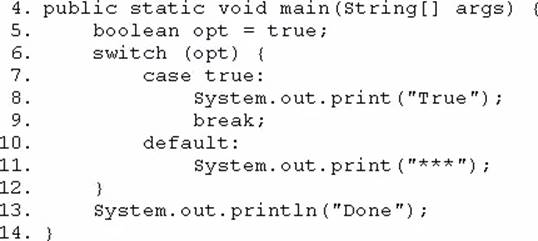Which modification enables the code fragment to print TrueDone?

22. Given the following main method: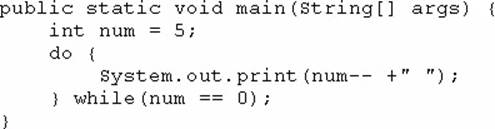What is the result?

23. Given the code fragment: What is the result?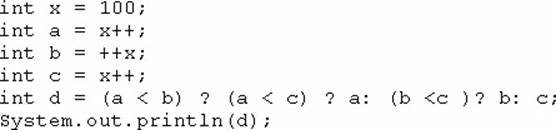24. Given: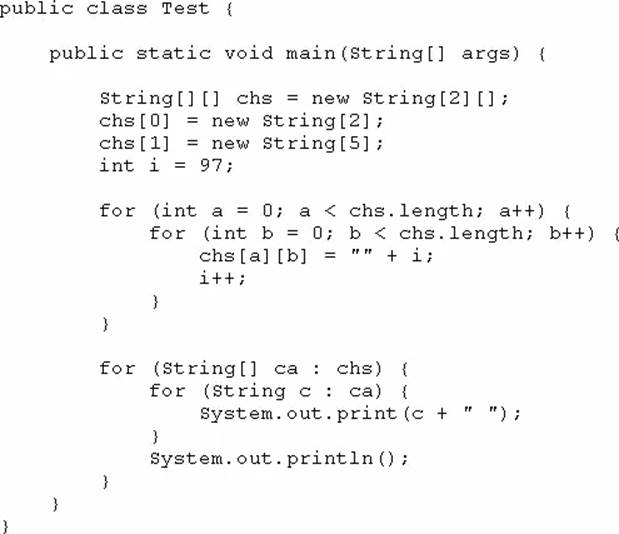What is the result?

25. Given the code fragment: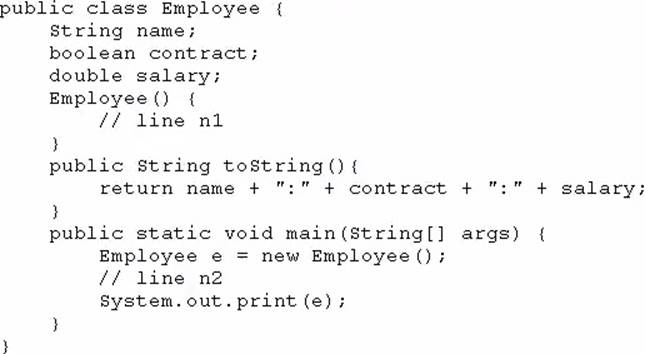Which two modifications, when made independently, enable the code to print joe:true: 100.0? (Choose two.)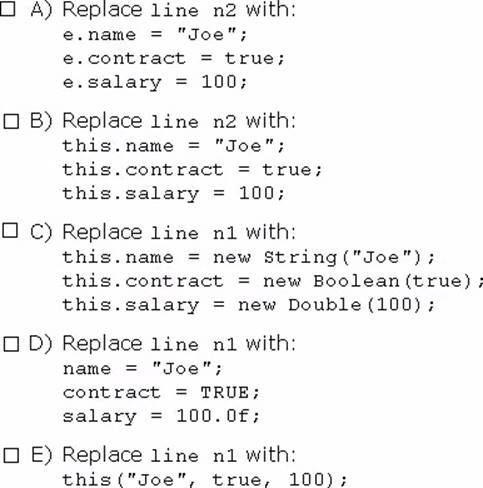26. Given the code fragment: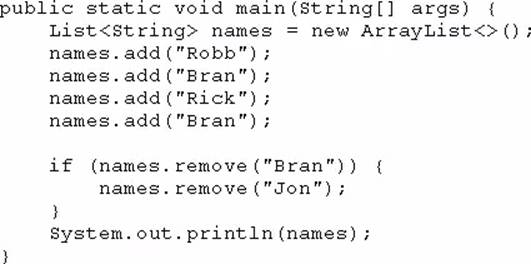What is the result?

27. Given: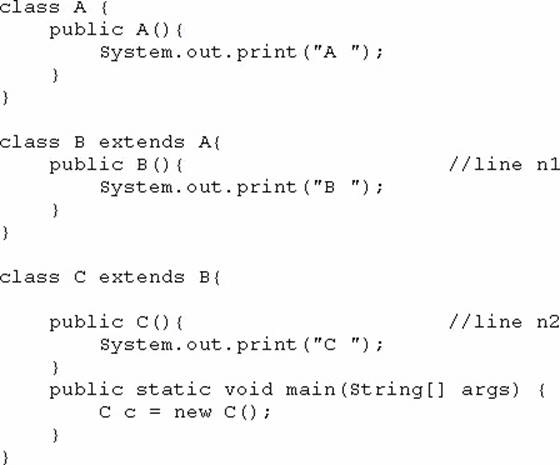What is the result?

28. Given: What is the result?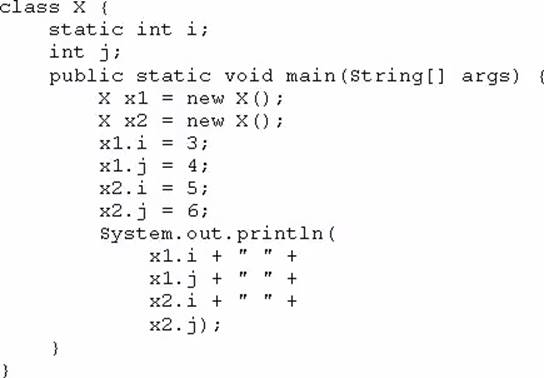29. Given the code fragment: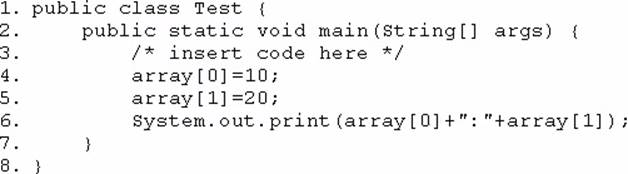Which code fragment, when inserted at line 3, enables the code to print 10:20?

30. Given the code fragment: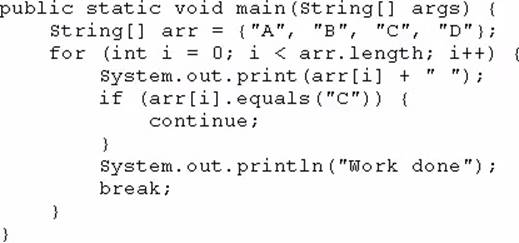What is the result?

31. Which three are advantages of the Java exception mechanism? (Choose three.)

32. Given the code from the Greeting.Java file: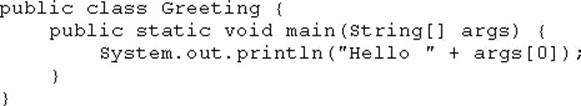Which set of commands prints Hello Duke in the console?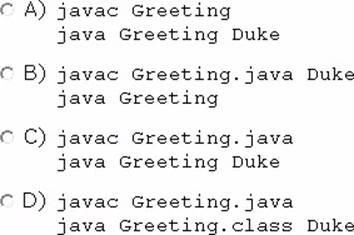33. Given: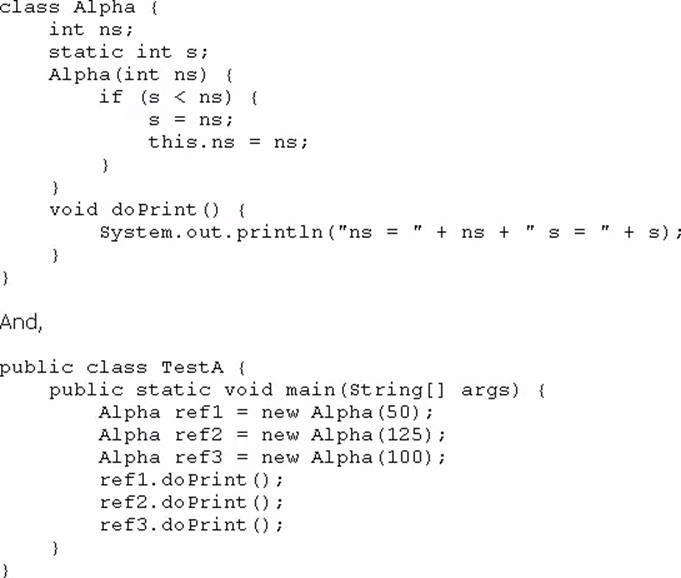What is the result?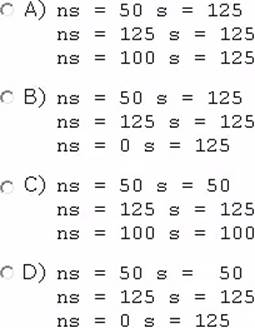34. Given the code fragment: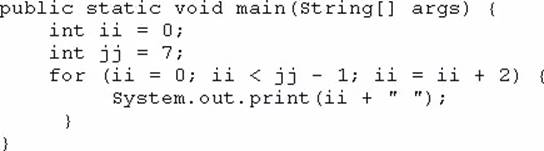What is the result?

35. Given the code fragment: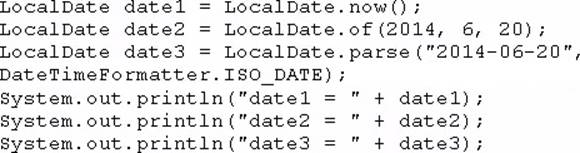Assume that the system date is June 20, 2014.

What is the result?

36. Given the code fragment: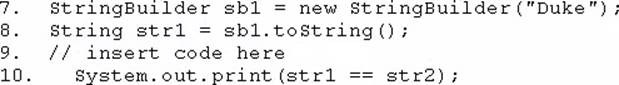Which code fragment, when inserted at line 9, enables the code to print true?

37. Given: What is the result?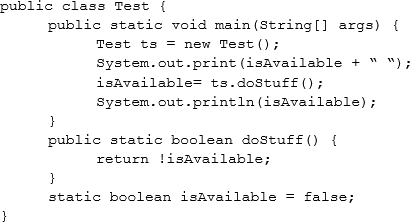38. Given the code fragment: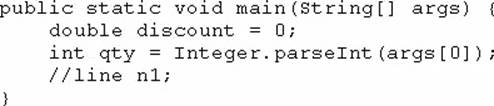And given the requirements:

If the value of the qty variable is greater than or equal to 90, discount = 0.5

If the value of the qty variable is between 80 and 90, discount = 0.2

Which two code fragments can be independently placed at line n1 to meet the requirements? (Choose two.)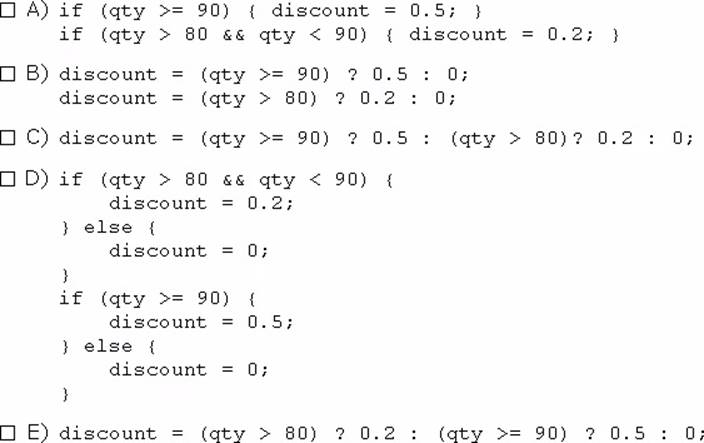39. Given: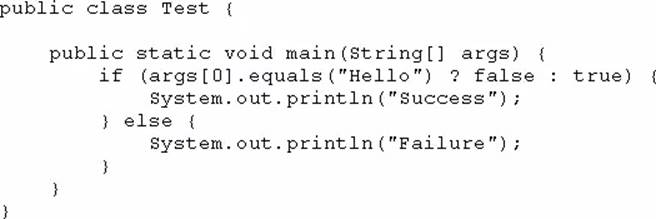And given the commands: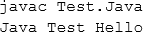What is the result?

40. Which three statements describe the object-oriented features of the Java language? (Choose three.)

41. Given the following code: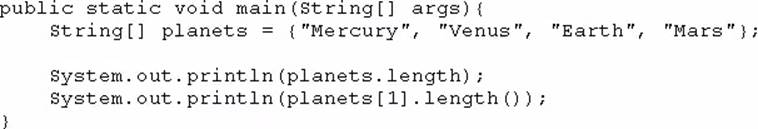What is the output?

42. You are developing a banking module. You have developed a class named ccMask that has a maskcc method.

Given the code fragment: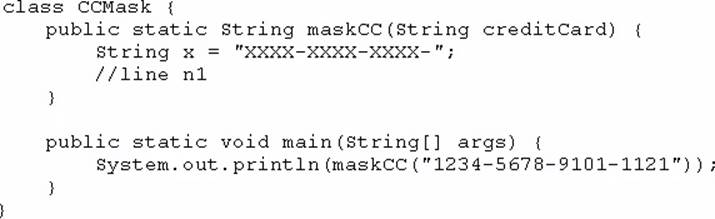You must ensure that the maskcc method returns a string that hides all digits of the credit card number except the four last digits (and the hyphens that separate each group of four digits).

Which two code fragments should you use at line n1, independently, to achieve this requirement? (Choose two.)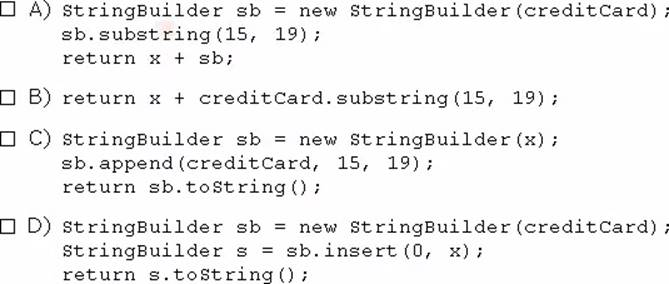43. Given: Acc.java: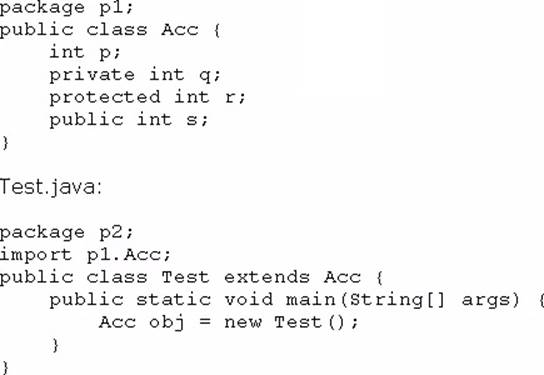Which statement is true?

44. Given: What is the result?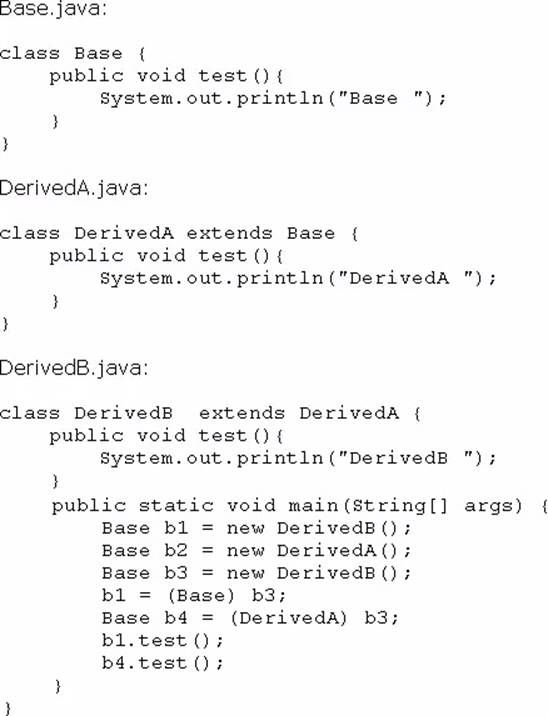45. Given the code fragment: What is the result?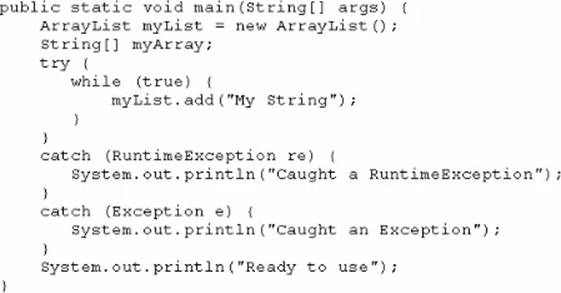46. Given: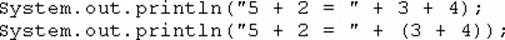What is the result?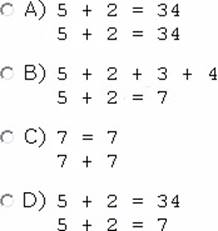47. Given the code fragments: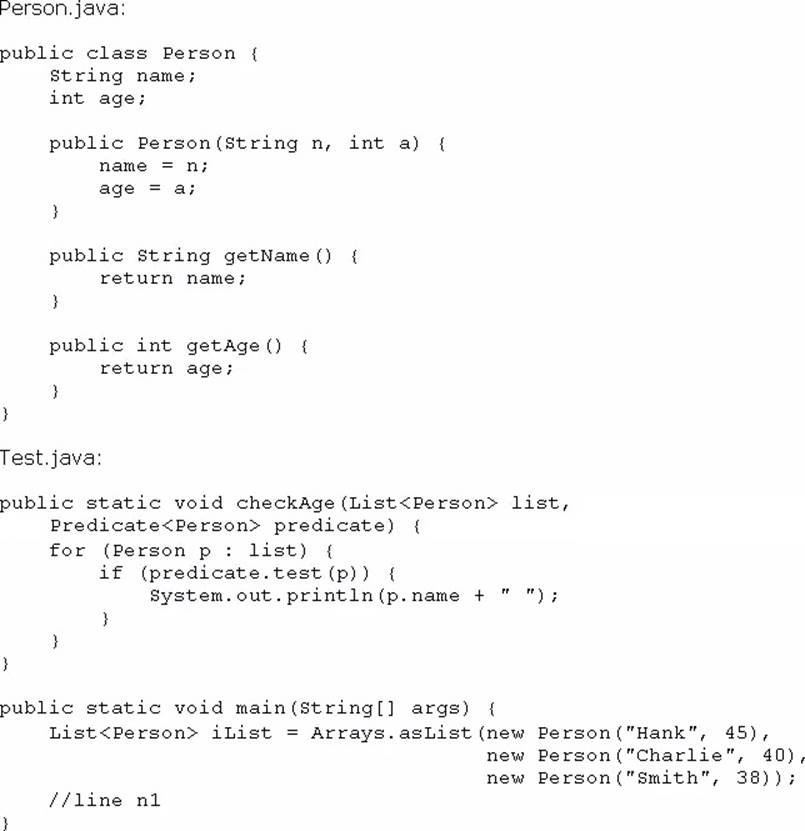Which code fragment, when inserted at line n1, enables the code to print Hank?

48. Given the code fragment: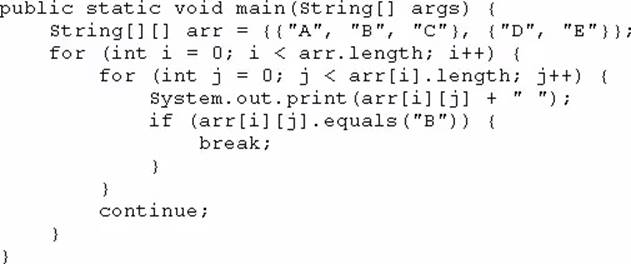What is the result?

49. Given the code fragment: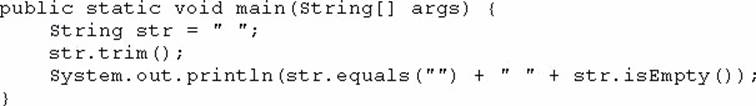What is the result?

50. Given the code fragment: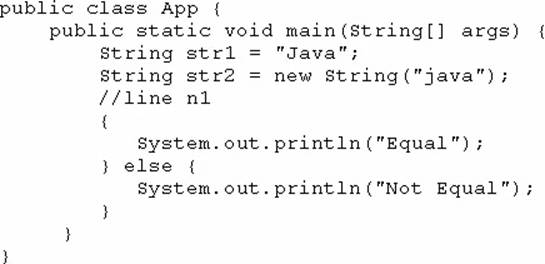Which code fragment, when inserted at line n1, enables the App class to print Equal?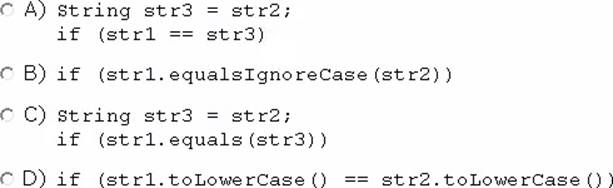51. Given the code fragment: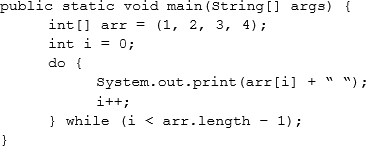What is the result?

52. Given the code fragment: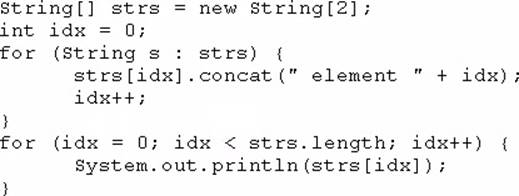What is the result?

53. Given: What is the result?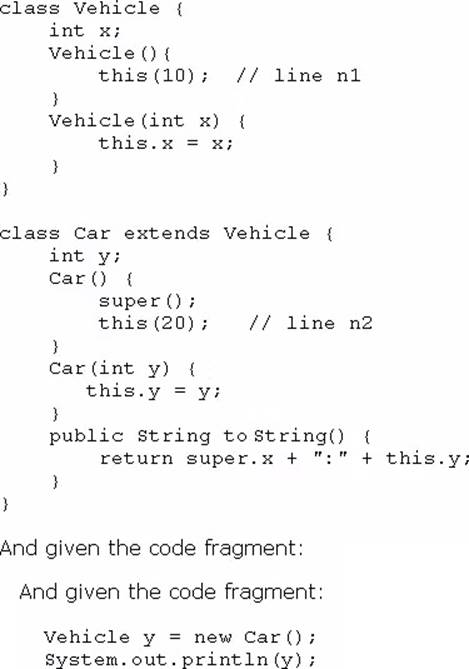54. Given the definitions of the MyString class and the Test class: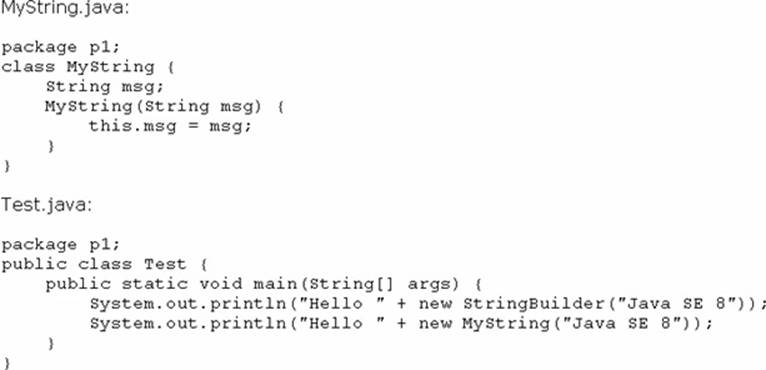What is the result?

55. Given the code fragment: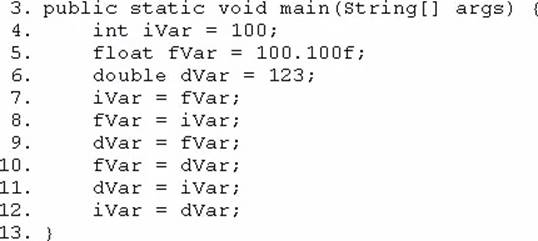Which three lines fail to compile? (Choose three.)

56. Given: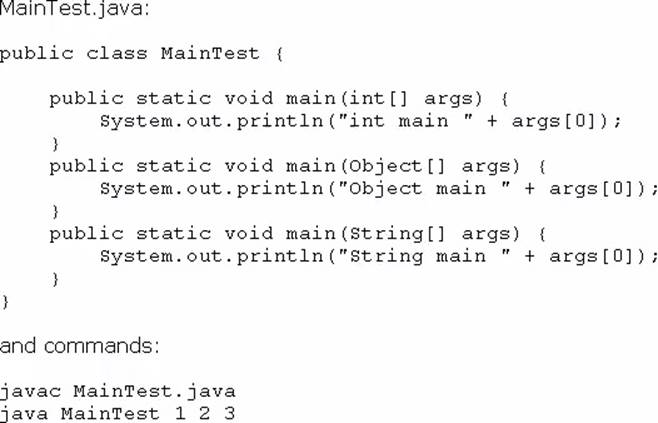What is the result?

57. Given the code fragment: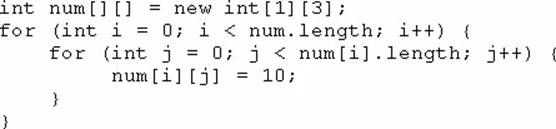Which option represents the state of the num array after successful completion of the outer loop?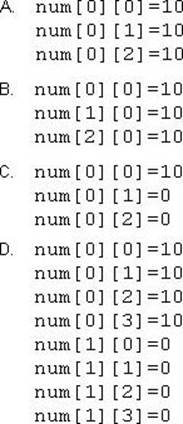58. Given the following code for a Planet object: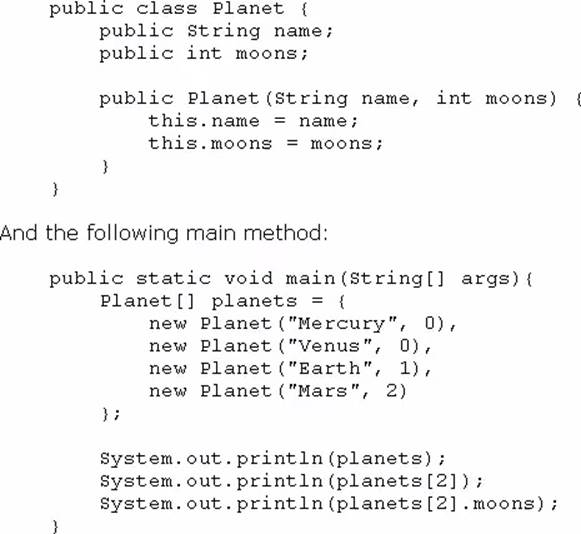What is the output?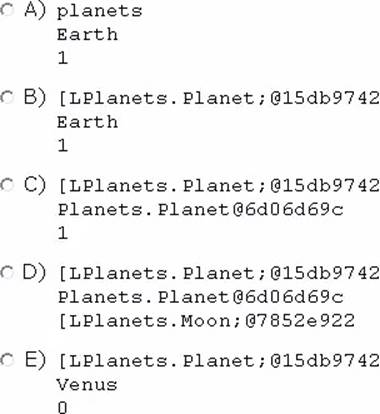59. You are asked to develop a program for a shopping application, and you are given the following information:

– The application must contain the classes Toy, EduToy, and ConsToy. The Toy class is the superclass of the other two classes.

– The int calculatePrice (Toy t) method calculates the price of a toy.

– The void printToy (Toy t) method prints the details of a toy.

Which definition of the Toy class adds a valid layer of abstraction to the class hierarchy?

60. Given the following code: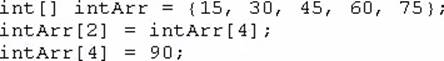What are the values of each element in intArr after this code has executed?

61. Given the following array: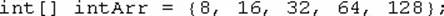Which two code fragments, independently, print each element in this array? (Choose two.)